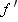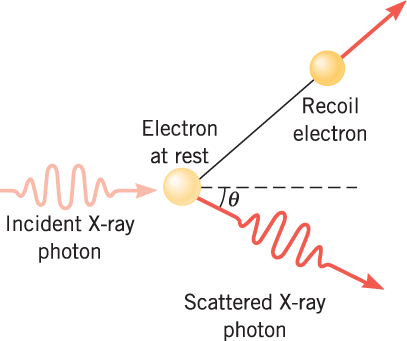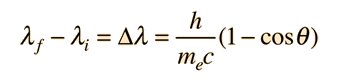• ×
السبت 6 يونيو 2020 | 05-28-2020
×

# تأثير كومبتون Compton-Effect

Although Einstein presented his photon model for the photoelectric effect in 1905, it was not until 1923 that the photon concept began to achieve widespread acceptance. It was then that the American physicist Arthur H. Compton (1892–1962) used the photon model to explain his research on the scattering of X-rays by the electrons in graphite. X-rays are high-frequency electromagnetic waves and, like light, they are composed of photons.

Figure 29-10 illustrates what happens when an X-ray photon strikes an electron in a piece of graphite. Like two billiard balls colliding on a pool table, the X-ray photon scatters in one direction after the collision, and the electron recoils in another direction. Compton observed that the scattered photon has a frequencythat is smaller than the frequency f of the incident photon, indicating that the photon loses energy during the collision. In addition, he found that the difference between the two frequencies depends on the angleat which the scattered photon leaves the collision. The phenomenon in which an X-ray photon is scattered from an electron, with the scattered photon having a smaller frequency than the incident photon, is called the Compton effect.Figure 29-10   In an experiment performed by Arthur H. Compton, an X-ray photon collides with a stationary electron. The scattered photon and the recoil electron depart the collision in different directions.Arthur H. Compton observed the scattering of x-raysfrom electrons in a carbon target and found scattered x-rays with a longer wavelength than those incident upon the target. The shift of the wavelength increased with scattering angle according to the Compton formula:Compton explained and modeled the data by assuming a particle (photon) nature for light and applying conservation of energy and conservation of momentum to the collision between the photon and the electron. The scattered photon has lower energy and therefore a longer wavelength according to the Planck relationship.

At a time (early 1920"s) when the particle (photon) nature of light suggested by thephotoelectric effect was still being debated, the Compton experiment gave clear and independent evidence of particle-like behavior. Compton was awarded the Nobel Prize in 1927 for the "discovery of the effect named after him".

## Compton Scattering Experiment.## Theoretical Analysis of Compton"s Experimental Observations

Compton used the Einstein’s concept of photon for the first time in the analysis of the observations in his experiments. A photon is a localized packet of energy. It may be considered as a particle of energy E0 given by Planck"s quantum hypothesis E0= hν and momentum p0 given by De Broglie relation p0 = h / λ where ν is the frequency and λ is the wavelength of the X ray photon.

The wavelength of the X ray photons is ≤1A0 and energy hν≥ 104 eV. Due to the high energy of the photons, the velocity acquired by the electrons is very high and comparable to the speed of light. Hence it is necessary to use relativistic expressions for the kinetic energy and momentum of the electrons.

The general equation for the total relativistic energy E in terms of rest mass m0 is given by$E= frac{m_0c^2}{sqrt{1-frac{ u^2}{c^2}}}$

Velocity of the photon is c, its energy E0 = hν is finite. Hence the rest mass of photon must be zero. Its energy is entirely kinetic, Momentum p0 of the photon is obtained from the general equation${E^2}= {c^2p^2}+ ({m_0c^2)}^2$$p=frac{E}{c}=frac{h u}{c}$

Momentum of photon p0 is given by p0 = h / λ

The interaction between incident X-ray photon and an electron in the target may be considered as a collision between two particles. Compared to the energy of the X-ray photons, the binding energy of the electrons in the target material is small and they may be treated as free electrons. X-ray Photon having total relativistic energy E0 and momentum p0 strikes a stationary electron having mass me and rest mass energy mec2. The incoming photon gives part of its energy to one of the loosely bound (almost-free electrons) in the outer shell of the atom or molecule in the stationary target This energy of photon is used to release and give kinetic energy to the electrons. After the collision the ejected electron recoils with kinetic energy T2 and momentum p2 in the direction making an angle ф. Its total energy E2is the sum of kinetic energy T2 and rest mass energy mec2. X-ray photon is scattered with the remaining total relativistic energy E1 and momentum p1in a direction making an angle θ with the original direction, This interaction is according to the conservation of linear momentum and total energy in inelastic scattering. As some energy of the scattered X- ray photons is lost (to the recoiling electron), the frequency ν of the scattered X-ray photon is decreased to ν’or its wavelength λ is increased to λ’. It is calculated using the relations as follows

According to the conservation of momentum${p_0} = {p_1} cos heta, +{p_2} cos Phi$$0= {p_1} sin heta,-{p_2} sinPhi$

Φ is eliminated by squaring and adding above two equations${p_2^2} = {p_0^2}+ {p_1^2}-2 {p_0 p_1} cos heta$

According to the conservation of total relativistic energy

E0 + mec2 = E1 + T2 + mec2

E0 − E1 = T2

hv − hv" = T2

However${p_0}=frac{E_0}{c}{p_1}=frac{E_1}{c}$

c(p0 − p1) = T2

Energy of the electron after collision is given by

E2 = T2 + mec2

E2 is also expressed by${E_2^2}= {c^2 p^2} +({m_e c^2})^2$

where the general equation E2 = c2p2 + (m0c2)2 is applied to the electron

Equating the above two expressions for energy of the electron$({T_2 + m_e c^2})^2= {c^2 p_2^2}+({m_e c^2})^2$${T_2^2}+ {2T_2 m_e c^2}= {c^2 p_2^2}$$frac{T_2^2}{c^2}+ {2T_2 m_e}= {p_2^2}$$(p_0-p_1)^2 + {2m_e c}(p_0-p_1)= {p_2^2} = {p_0^2}+ {p_1^2-2 p_0 p_1} cos heta$

mec(p0 − p1) = (p0p1)(1 − cosθ)$frac{1}{p_1}-frac{1}{p_0} =frac{1}{m_e c}(1-cos heta)=frac{1}{m_e c}(2 sin^2⁡frac{ heta}{2})$

Using algebraic relations to eliminate variables Compton arrived at the following relationship between the shift in wavelength$Deltalambda =(lambda^$ and the scattering angle θ in terms of constant parameters as follows$Deltalambda =lambda^$$lambda,_c = frac{h}{m_ec}= 2.43 x 10^{-12}m=0.0243 {A^0}$$lambda,$ is the initial wavelength of the X-ray photon$lambda^$ is the wavelength after scattering of the X-ray photon$lambda,_c$ is the Compton wavelength

h is the Planck constant

me is the mass of the electron

c is the speed of light

θ is the scattering angle of the X-ray photon.

It is known as the Compton equation. From the formula, theoretical value of the wavelength$lambda^$ for scattering at θ = 900 comes out to be 0.0733 nm. It is consistent with the experimental value 0.0731 nm (refer to the table)

Compton Shift

The quantity$lambda,_c = h/m_e c$ is called as the Compton wavelength of the electron. Its value is equal to 2.43×10 − 12 m.

The wavelength shift$Deltalambda =lambda^$ is called as the Compton Shift.

The Compton Shift$Deltalambda =(lambda^$ varies with the scattering angle$heta,$ of photon as follows

It has Minimum value Δλ = zero (for θ = 0°)

when the incident photon is not deflected from its path

It is called as a “Grazing collision” of photon with electron

It has Maximum value$Deltalambda,= 2h/{m_e c}$ = Twice the Compton wavelength (for θ= 180°).

When the incident photon reverses its direction

It is called as a “Head-on collision” of photon with electron

From the graphs it is seen that in addition to the shifted wavelength$lambda^$ an unmodified wavelength$lambda,$ also is present. It is due to the scattering of X-ray photon from tightly bound electrons. In that case, not a single loose or free electron but the entire atom is involved in the interaction with photon and it recoils. In the formula for$lambda,_c$mass of atom MA (instead of mass of electronme) is to be used. MA > > me. Hence,$Deltalambda,$ is a minute, almost negligible quantity i.e. wavelength$lambda,$ remains unaffected after scattering.

Similar reasoning applies if light photons in the visible range are used. They have longer wavelengths of the order of 500 nm. Their energies are far too smaller than X-rays and not sufficient to overcome the binding energies of even the loosely bound electrons. The wavelength shift$(lambda^$ is very very small in comparison to their wavelength. By using them it is difficult to detect minute wavelength shift$(lambda^$.On the contrary, X-rays have short wavelengths of the order of 0.1 nm. Their use makes the measurement of minute wavelength shift (of less than 0.1 nm) comparatively easy. Hence X ray photons were found to be more appropriate to demonstrate the scattering between photons and electrons and confirm the particle nature of light.

## 1.4.3 The Compton Effect

Compton scattering is a type of inelastic scattering that high energy X-rays, gamma rays undergo in matter. When a X ray or gamma ray photon collides with an electron in matter, part of its energy is transferred to the electron, which recoils and is ejected from its atom (atom becomes ionized). X ray or gamma ray is scattered with the remaining energy. Due to the "degradation" or a decrease in energy, its wavelength is increased. It is called as the Compton effect.

The relationship between the shift in wavelength$(lambda^$ and the scattering angle θ, called as the Compton shift is given by$Delta,lambda, = lambda^$$lambda_c, = frac{h}{m_0c} = 0.0243x10^{-12}m = 0.0243 A^0$

The Compton shift depends upon the angle of scattering θ,. Wavelength λ’ of the scattered X ray photon depends upon the angle of scattering θ and also the mass of the recoiling electron

بواسطة :
05-29-2016 12:00 صباحاً  0  0  2.3K
التعليقات ( 0 )